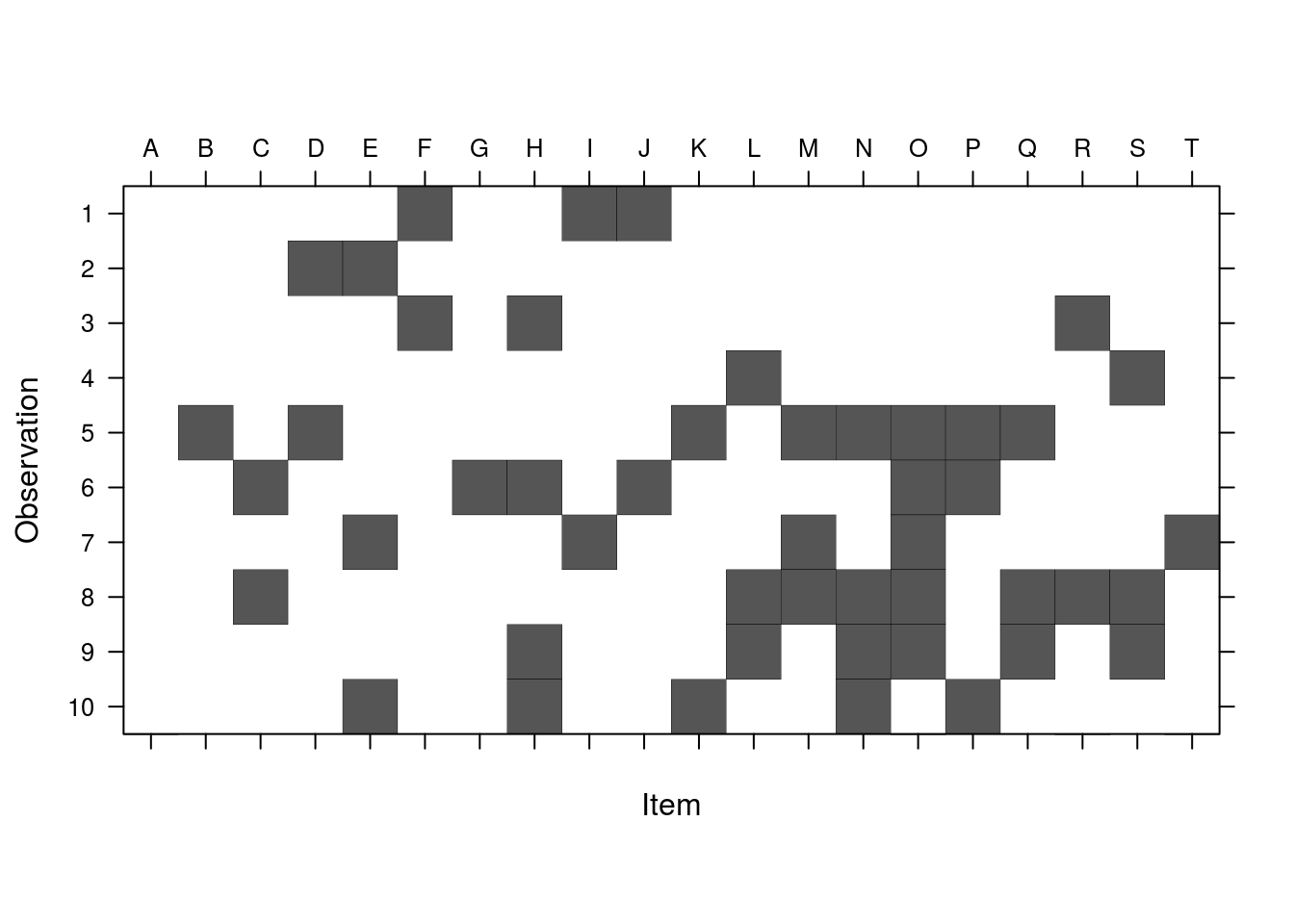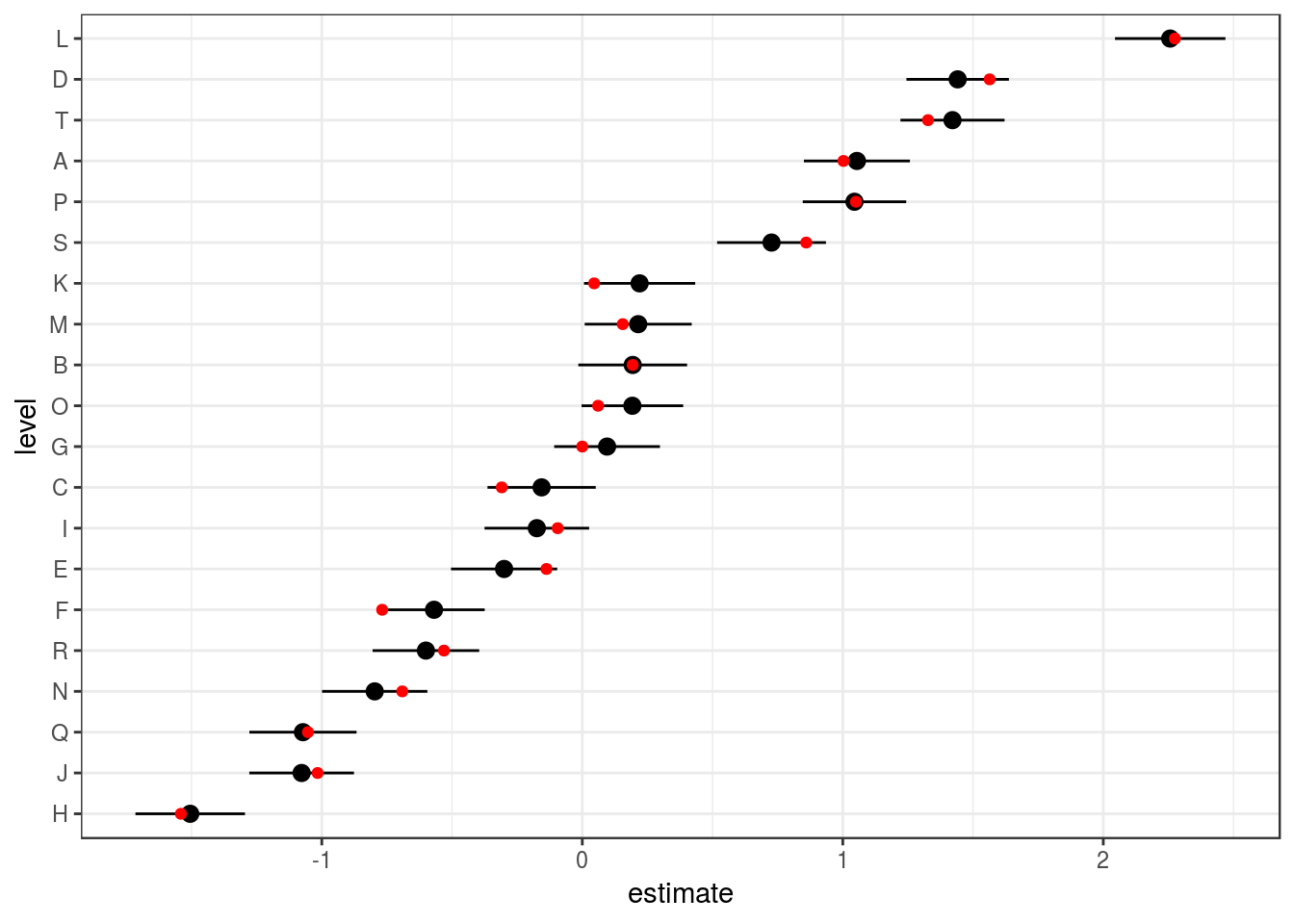Consider a situation where we have multiple items of some sort which have associated effects, but we can’t association observations with single items; instead, each observation is associated with a group of items. (Two examples which have come up are (1) authorship of papers and (2) hockey players on a team.) These are not quite identical to “multi-membership models” (I think), although similar techniques to those shown here could work for multi-membership models.

Load packages (we don’t really need anything beyond lme4; the rest are for convenience/drawing pictures).

library(lme4)
library(broom.mixed)
library(ggplot2); theme_set(theme_bw())
library(Matrix)

Construct a simulated example: first, simulate the design (structure).

nm <- 20
nobs0 <- 500
set.seed(101)
## choose items for observations
W <- matrix(rbinom(nobs0*nm, prob=0.25, size=1),nrow=nobs0,ncol=nm)
dimnames(W) <- list(NULL,LETTERS[seq(nm)])
W[1:5,]
##      A B C D E F G H I J K L M N O P Q R S T
## [1,] 0 0 0 0 0 1 0 0 1 1 0 0 0 0 0 0 0 0 0 0
## [2,] 0 0 0 1 1 0 0 0 0 0 0 0 0 0 0 0 0 0 0 0
## [3,] 0 0 0 0 0 1 0 1 0 0 0 0 0 0 0 0 0 1 0 0
## [4,] 0 0 0 0 0 0 0 0 0 0 0 1 0 0 0 0 0 0 1 0
## [5,] 0 1 0 1 0 0 0 0 0 0 1 0 1 1 1 1 1 0 0 0
table(rowSums(W))
##
##   1   2   3   4   5   6   7   8   9  10  11
##  11  40  63  85 106  85  52  37  11   7   3

The first 10 observations:

image(Matrix(W),ylim=c(1,10),sub="",ylab="Observation",
xlab="Item",
## draw tick labels at top
scales=list(at=1:20,x=list(labels=colnames(W),
alternating=2)))Since we chose which items/individuals to include for each observation randomly and independently (Bernoulli with probability 0.25), we have a highly variable number of individuals present for different observations (1-11). This would be realistic for some examples (authorship), unrealistic for others (hockey) … I don’t think it really makes much difference computationally (statistically, having some observations with a single member must make estimation more powerful …) The 0/1 matrix (indicator variable for whether item $$i$$ is included in observation $$j$$) is convenient, and will turn out to be the form we need for inclusion in the model. It should be fairly straightforward to convert other forms (e.g. a list of sets of items associated with each observation) to this form …

Now simulate the response variable.

b <- rnorm(nm)  ## item-level effects
beta <- c(1,2)
M <- data.frame(x=runif(nobs0))
X <- model.matrix(~x, data=M)
## n.b. get in trouble if we don't add residual error
## (theta is scaled relative to residual error)
## here, use theta=sigma=1
M$y <- c(X %*% beta) + c(W %*% b) +rnorm(nobs0,sd=1) Fit (see ?modular): ## helpful to specify a factor with the right levels: ## actual values are unimportant since we will specify Zt/Ztlist directly M$fake <- rep(LETTERS[seq(nm)],length.out=nobs)
lmod <- lFormula(y~x+(1|fake), data=M)
lmod$reTrms$Zt <- lmod$reTrms$Ztlist[] <- Matrix(t(W))
devfun <- do.call(mkLmerDevfun, lmod)
opt <- optimizeLmer(devfun)
m1 <- mkMerMod(environment(devfun), opt, lmod$reTrms, fr = lmod$fr)

Results look OK (correct fixed effects, item and residual variance estimated):

m1
## Linear mixed model fit by REML ['lmerMod']
## REML criterion at convergence: 1506.767
## Random effects:
##  Groups   Name        Std.Dev.
##  fake     (Intercept) 0.9580
##  Residual             0.9944
## Number of obs: 500, groups:  fake, 20
## Fixed Effects:
## (Intercept)            x
##      0.8534       2.2106

The conditional modes by item look good:

dd <- tidy(m1, effects="ran_vals")
dd <- transform(dd, level=reorder(level,estimate))
truth <- data.frame(level=LETTERS[seq(nm)],estimate=b)
ggplot(dd,aes(x=level,y=estimate))+
geom_pointrange(aes(ymin=estimate-2*std.error,
ymax=estimate+2*std.error))+coord_flip()+
geom_point(data=truth,colour="red")sos::findFn("{multiple membership}") ## GPvam, R2MLwiN, RealVAMS, ...
• r-sig-mixed-models posts (here, here)
• mm(), mmc() in the brms package
• mult.memb() in the MCMCglmm() package

## function encapsulation

Suppose we have a real data frame M and a (sparse) weight matrix W (or? a list L of weight vectors?

##' @param formula mixed model formula
##' @param data data frame (possibly but not necessarily containing factors
##' @param memb_mat list of weights matrices  with which to replace Zt components
lmer_multimemb <- function(formula,data,memb_mat=list(),...) {
## FIXME: pass ... through appropriately
## FIXME: test dimensions
mnms <- names(memb_mat)
fb <- findbars(formula)
gvars <- vapply(fb, function(x) deparse(x[]), character(1))
Ztlist <- list()
for (i in seq_along(fb)) {
fbnm <- deparse(fb[[i]])
## find corresponding random-effects term
w <- which(mnms==gvars[i])
if (length(w)>0) {
M <- Matrix::Matrix(memb_mat[[w]])
## extract LHS (effect)
form <- as.formula(substitute(~z,list(z=fb[[i]][])))
## construct model matrix & compute Khatri-Rao product
X <- model.matrix(form,data=data)
Zt <- Matrix::KhatriRao(t(M),t(X),make.dimnames=TRUE)
## FIXME: mess with names?
Ztlist[[fbnm]] <- Zt
## if necessary, add factor to data
if (!m %in% names(data)) {
## if the factor has non-trivial ordering, it should be included
## in the data.  Do we have to worry about ordering of Z? test!
data[[gvars[i]]] <- factor(colnames(memb_mat[[w]]))
}
} ## if  (length(w)>0)
} ## for i in seq(fb)
## call lFormula  (FIXME: allow glFormula)
lmod <- lFormula(formula,data=data)
## substitute new Ztlist elements
for (m in names(Ztlist)) {
lmod$reTrms$Ztlist[[m]] <- Ztlist[[m]]
}
lmod$reTrms$Zt <- do.call(rbind,lmod$reTrms$Ztlist)
## finish fitting
devfun <- do.call(mkLmerDevfun, lmod)
opt <- optimizeLmer(devfun)
m1 <- mkMerMod(environment(devfun), opt, lmod$reTrms, fr = lmod$fr)
return(m1)
}

This seems to work …

lmer_multimemb(formula=y ~ x + (1|team),
data=M,
memb_mat=list(team=W))
## Linear mixed model fit by REML ['lmerMod']
## REML criterion at convergence: 1506.767
## Random effects:
##  Groups   Name        Std.Dev.
##  team     (Intercept) 0.9580
##  Residual             0.9944
## Number of obs: 500, groups:  team, 20
## Fixed Effects:
## (Intercept)            x
##      0.8534       2.2106

## Data formats

We want to allow users to conveniently transform whatever data formats they have to a sparse incidence matrix.

### memberships in multiple columns

Maybe it’s in multiple columns (as in this example from Harold Doran):

tmp <- data.frame(id = 1:5,
tch1 = c(10,10,11,11,12),
tch2 = c(77,15,NA,10,11),
tch3 = c(NA, NA, 77, 12, 10),
y = rnorm(5), x = rnorm(5))
## list of teachers
all_tch <- with(tmp,sort(unique(na.omit(c(tch1,tch2,tch3)))))
## empty (sparse) matrix with dimnames
W <- Matrix(0,nrow=nrow(tmp),ncol=length(all_tch),
dimnames=list(as.character(tmp$id),as.character(all_tch))) ## melt all teacher columns to long/combined format all_prs0 <- na.omit(reshape2::melt(tmp[,1:4],id="id")) all_prs1 <- all_prs0[,c("id","value")] ## convert to numeric indices all_prs2 <- all_prs1 all_prs2[] <- lapply(all_prs2,function(x) as.numeric(factor(x))) ## convert to two-column matrix all_prs2 <- as.matrix(all_prs2) W[all_prs2] <- 1 ## weights ## row_ID, col_ID, weight as three columns As pointed out by Robyn Wimmers, reshape2::dcast is handy if your data are in a three-column format (row_label, col_label, weight). It’s marginally less efficient because it initially generates a dense matrix. ## convert from the format we have above all_prs3 <- all_prs1 all_prs3[] <- lapply(all_prs3, factor) all_prs3$wts <- 1
## now data frame -> matrix
W0 <- reshape2::dcast(all_prs3,id~value, value.var="wts", fill=0)
idvars <- W0\$id
W <- Matrix(as.matrix(W0[,-1])) ## drop 'id' column and sparsify
rownames(W) <- as.character(idvars)

Session info:

sessionInfo()
## R Under development (unstable) (2019-03-04 r76196)
## Platform: x86_64-pc-linux-gnu (64-bit)
## Running under: Ubuntu 16.04.6 LTS
##
## Matrix products: default
## BLAS: /usr/local/lib/R/lib/libRblas.so
## LAPACK: /usr/local/lib/R/lib/libRlapack.so
##
## locale:
##   LC_CTYPE=en_CA.UTF8       LC_NUMERIC=C
##   LC_TIME=en_CA.UTF8        LC_COLLATE=en_CA.UTF8
##   LC_MONETARY=en_CA.UTF8    LC_MESSAGES=en_CA.UTF8
##   LC_PAPER=en_CA.UTF8       LC_NAME=C
##  LC_MEASUREMENT=en_CA.UTF8 LC_IDENTIFICATION=C
##
## attached base packages:
##  stats     graphics  grDevices utils     datasets  methods   base
##
## other attached packages:
##  spdep_1.0-2       sf_0.7-3          spData_0.3.0      sp_1.3-1
##  ggplot2_3.1.0     broom.mixed_0.2.4 lme4_1.1-21       Matrix_1.2-16
##
## loaded via a namespace (and not attached):
##   gtools_3.8.1      tidyselect_0.2.5  xfun_0.5
##   TMB_1.7.15        purrr_0.3.1       reshape2_1.4.3
##   splines_3.6.0     lattice_0.20-38   expm_0.999-3
##  colorspace_1.4-0  generics_0.0.2    htmltools_0.3.6
##  yaml_2.2.0        rlang_0.3.1       e1071_1.7-0.1
##  nloptr_1.2.1      pillar_1.3.1      glue_1.3.0
##  withr_2.1.2.9000  DBI_1.0.0         plyr_1.8.4
##  stringr_1.4.0     munsell_0.5.0     gtable_0.2.0
##  coda_0.19-2       evaluate_0.13     labeling_0.3
##  knitr_1.21        class_7.3-15      broom_0.5.1
##  Rcpp_1.0.0        backports_1.1.3   scales_1.0.0
##  classInt_0.3-1    gdata_2.18.0      deldir_0.1-16
##  digest_0.6.18     gmodels_2.18.1    stringi_1.3.1
##  dplyr_0.8.0.9001  grid_3.6.0        LearnBayes_2.15.1
##  tools_3.6.0       magrittr_1.5      lazyeval_0.2.1
##  tibble_2.0.1      crayon_1.3.4      tidyr_0.8.3
##  pkgconfig_2.0.2   MASS_7.3-51.2     assertthat_0.2.0
##  minqa_1.2.4       rmarkdown_1.11    R6_2.4.0
##  boot_1.3-21       units_0.6-2       nlme_3.1-137
##  compiler_3.6.0